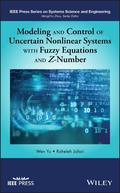John Wiley & Sons Modeling and Control of Uncertain Nonlinear Systems with Fuzzy Equations and Z-NumberAn original, systematic-solution approach to uncertain nonlinear systems control and modeling using .. Product #: 978-1-119-49155-2 Regular price: \$85.89 \$85.89 Auf Lager

# Modeling and Control of Uncertain Nonlinear Systems with Fuzzy Equations and Z-Number

IEEE Series on Systems Science and Engineering1. Auflage September 2019
208 Seiten, Hardcover
Wiley & Sons Ltd

ISBN: 978-1-119-49155-2
John Wiley & Sons

Jetzt kaufen

### Preis: 91,90 €

Preis inkl. MwSt, zzgl. Versand

An original, systematic-solution approach to uncertain nonlinear systems control and modeling using fuzzy equations and fuzzy differential equations

There are various numerical and analytical approaches to the modeling and control of uncertain nonlinear systems. Fuzzy logic theory is an increasingly popular method used to solve inconvenience problems in nonlinear modeling. Modeling and Control of Uncertain Nonlinear Systems with Fuzzy Equations and Z-Number presents a structured approach to the control and modeling of uncertain nonlinear systems in industry using fuzzy equations and fuzzy differential equations.

The first major work to explore methods based on neural networks and Bernstein neural networks, this innovative volume provides a framework for control and modeling of uncertain nonlinear systems with applications to industry. Readers learn how to use fuzzy techniques to solve scientific and engineering problems and understand intelligent control design and applications. The text assembles the results of four years of research on control of uncertain nonlinear systems with dual fuzzy equations, fuzzy modeling for uncertain nonlinear systems with fuzzy equations, the numerical solution of fuzzy equations with Z-numbers, and the numerical solution of fuzzy differential equations with Z-numbers. Using clear and accessible language to explain concepts and principles applicable to real-world scenarios, this book:
* Presents the modeling and control of uncertain nonlinear systems with fuzzy equations and fuzzy differential equations
* Includes an overview of uncertain nonlinear systems for non-specialists
* Teaches readers to use simulation, modeling and verification skills valuable for scientific research and engineering systems development
* Reinforces comprehension with illustrations, tables, examples, and simulations

Modeling and Control of Uncertain Nonlinear Systems with Fuzzy Equations and Z-Number is suitable as a textbook for advanced students, academic and industrial researchers, and practitioners in fields of systems engineering, learning control systems, neural networks, computational intelligence, and fuzzy logic control.

List of Figures xi

List of Tables xiii

Preface xv

1 Fuzzy Equations 1

1.1 Introduction 1

1.2 Fuzzy Equations 1

1.3 Algebraic Fuzzy Equations 3

1.4 Numerical Methods for Solving Fuzzy Equations 5

1.4.1 Newton Method 5

1.4.2 Steepest Descent Method 7

1.4.4 Ranking Method 9

1.4.5 Intelligent Methods 10

1.4.5.1 Genetic Algorithm Method 10

1.4.5.2 Neural Network Method 11

1.4.5.3 Fuzzy Linear Regression Model 14

1.5 Summary 20

2 Fuzzy Differential Equations 21

2.1 Introduction 21

2.2 Predictor-Corrector Method 21

2.4 Euler Method 23

2.5 Taylor Method 25

2.6 Runge-Kutta Method 25

2.7 Finite Difference Method 26

2.8 Differential Transform Method 28

2.9 Neural Network Method 29

2.10 Summary 36

3 Modeling and Control Using Fuzzy Equations 39

3.1 Fuzzy Modeling with Fuzzy Equations 39

3.1.1 Fuzzy Parameter Estimation with Neural Networks 45

3.1.2 Upper Bounds of the Modeling Errors 48

3.2 Control with Fuzzy Equations 52

3.3 Simulations 59

3.4 Summary 67

4 Modeling and Control Using Fuzzy Differential Equations 69

4.1 Introduction 69

4.2 Fuzzy Modeling with Fuzzy Differential Equations 69

4.3 Existence of a Solution 72

4.4 Solution Approximation using Bernstein Neural Networks 79

4.5 Solutions Approximation using the Fuzzy Sumudu Transform 83

4.6 Simulations 85

4.7 Summary 99

5 System Modeling with Partial Differential Equations 101

5.1 Introduction 101

5.2 Solutions using Burgers-Fisher Equations 101

5.3 Solution using Wave Equations 106

5.4 Simulations 109

5.5 Summary 117

6 System Control using Z-numbers 119

6.1 Introduction 119

6.2 Modeling using Dual Fuzzy Equations and Z-numbers 119

6.3 Controllability using Dual Fuzzy Equations 124

6.4 Fuzzy Controller 128

6.5 Nonlinear System Modeling 131

6.6 Controllability using Fuzzy Differential Equations 131

6.7 Fuzzy Controller Design using Fuzzy Differential Equations and Z-number 135

6.8 Approximation using a Fuzzy Sumudu Transform and Z-numbers 138

6.9 Simulations 139

6.10 Summary 151

References 153

Index 167
Wen Yu, PhD, is Professor at CINVESTAV-IPN (National Polytechnic Institute), Mexico City, Mexico. He is Associate Editor of IEEE Transactions on Cybernetics, Neurocomputing, and Journal of Intelligent and Fuzzy Systems. Dr. Yu is a member of the Mexican Academy of Sciences.

Raheleh Jafari is a postdoctoral research fellow at Centre for Artificial Intelligence Research (CAIR), University of Agder, Grimstad, Norway. She is on the editorial board of the Journal of Intelligent and Fuzzy Systems, and served as a reviewer in various journals and conferences. Her research interest is in the field of artificial intelligence, fuzzy control, machine learning, nonlinear systems, neural networks, and fuzzy engineering.